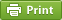Werner's Blog — Opinion, Analysis, Commentary
The multi-agent joint production problem

Many economic models are focused on competition among firms, each maximizing profits and interacting with each other in a market place. Economic models in which firms interact with each other in the production process (as opposed to the market for goods or intermediates) are not very common. However, they are useful for example in the context of fisheries economics where effort from one fishing boat affects the overall harvest and thus the stock available to all other fishing boats. The model below is taken from this context, presented in a UBC workshop by eminent fisheries economist Martin Smith. I am distilling this model for the benefit of my students who may find that this model has relevance in non-fisheries applications as well.

Consider $$n$$ economic agents that produce a common good with output $$Y=F(Q)$$ and joint effort $Q=\sum_{i=1}^n q_i$ where each agent receives a share of output equal to the share of effort $$s_i\equiv q_i/Q$$, and maximizes profit $\pi_i=p\cdot (q_i/Q)\cdot F(Q) - c_i\cdot q_i$ and faces constant marginal cost $$c_i$$. The shape of the production function $$F$$ is crucial. In fisheries economics the function is backward-bending as increased effort eventually starts depleting the stock; for example, $$F(Q)=u\cdot Q - v\cdot Q^2$$ with $$a>0,b>0$$. In other applications, expanding effort will typically face diminishing returns, such as $$F(Q)=u\cdot\sqrt{Q}$$; or more generally $$F(Q)=u\cdot Q^{1/(1+\theta)}$$ with $$\theta>0$$. The first-order condition for a profit maximum yields that optimal effort share is $s_i =\frac{Y/Q-c_i/p}{n\cdot(Y/Q-F_Q)}$ where $$F_Q\equiv\partial F/\partial Q$$ is the marginal product and $$Y/Q$$ is the average product. The optimal amount of effort thus depends on both marginal and average product, which is a departure from the more common framework where everything depends on the margin.

What kind of economic situations would correspond to such a model? Consider producing a video game where a group of programmers gets together and commit different amounts of time to the project, and agree that they will split the proceeds in proportionate share of their time contribution. Making the video game more and more complex will eventually lead to diminishing returns. So this may be a situation where joint production problem may arise. Each contributor is unable to observe others' marginal cost, and thus there is a type of Cournot equilibrium that emerges.

Let us continue solving the model. Introducing $\bar{c}=(1/n)\sum_{i=1}^n c_i$ as the average cost of effort, summing over all agents reveals that $\frac{p}{\bar{c}}=\frac{1}{(1/n)\cdot F_Q+(1-1/n)\cdot(Y/Q)}$ The above equation shows how the marginal product and average product are weighted, with the smaller weight going to the marginal product when $$n>2$$. We also need to pin down demand. Assume demand is linear so that $Y=a-b\cdot p$ We also assume that output is diminishing in joint effort so that $$F(Q)=u\cdot\sqrt{Q}$$. Then $$Y/Q-F_Q=Y/(2Q)$$. Further introduce the short-hand notation $$m\equiv 1-1/(2n)$$. Now $Q=\left[\frac{a}{u+(b\cdot\bar{c})/(m\cdot u)}\right]^2$ and therefore $p=\bar{c}\frac{\sqrt{Q}}{u\cdot m} = \frac{a\cdot\bar{c}}{m\cdot u^2+b\cdot\bar{c}}$ Finally, going back to the effort contribution, $s_i =\frac{1}{n}+2 m\left(\frac{\bar{c}-c_i}{\bar{c}}\right)$ The contribution share equation looks a lot like the outcome of a standard Cournot model. But note that the demand parameters or the scale parameter $$u$$ do not feature in the share equation. It is just the number of participants and the cost distribution that matters for determining relative effort contributions. However, the demand and scale parameters matter for determining overall effort.

The share equation has some interesting implications. In order for the effort share to be less than one, it must hold that $\frac{c_i}{\bar{c}}> \frac{n}{2n-1}$ So the cost advantage of any one contributor cannot be too large, or this contributor will take up all of the effort. This imposes an important constraint on the cost distribution. Consider the case of $$n=2$$ and assume $$c_1 < c_2$$, so contributor 1 is less costly than contributor 2. Then for both two contribute it must hold that $$c_1 > c_2/2$$: the higher-cost contributor cannot be more than twice as costly as the lower-cost contributor. Eventually this cost distribution will shrink. The last contributor to join cannot have costs that are much above the previous average $$\bar{c}_{n-1}$$; specifically, it must hold that $1<\frac{c_n}{\bar{c}_{n-1}}<\frac{2n-2}{2n-3}$ In essence, collaborators on a joint production problem need to have relatively similar costs, or the joint production will not happen and instead the lowest-cost provider produces all of the output.

What are the takeaways from the above model? Joint production doesn't all that different from Cournot competition. After all, there is a Nash equilibrium embedded in the model. The lowest-cost contributors get a larger share of the overall pie, just like low-cost producers get a larger market share in the conventional Cournot model. Where the model gets interesting, perhaps, is when the collaboration function $$F(Q)$$ is inverse-U shaped as in fisheries economics. To read more on this topic, please read Banzhaf, Liu, Smith, and Asche: Non-Parametric Tests of the Tragedy of the Commons, NBER Working Paper 26398.

Posted on Tuesday, January 12, 2021 at 15:00 — #EconomicsRecent Blog Entries

Topics

Months

Subscribe to RSS feed© 2023  Prof. Werner Antweiler, University of British Columbia.